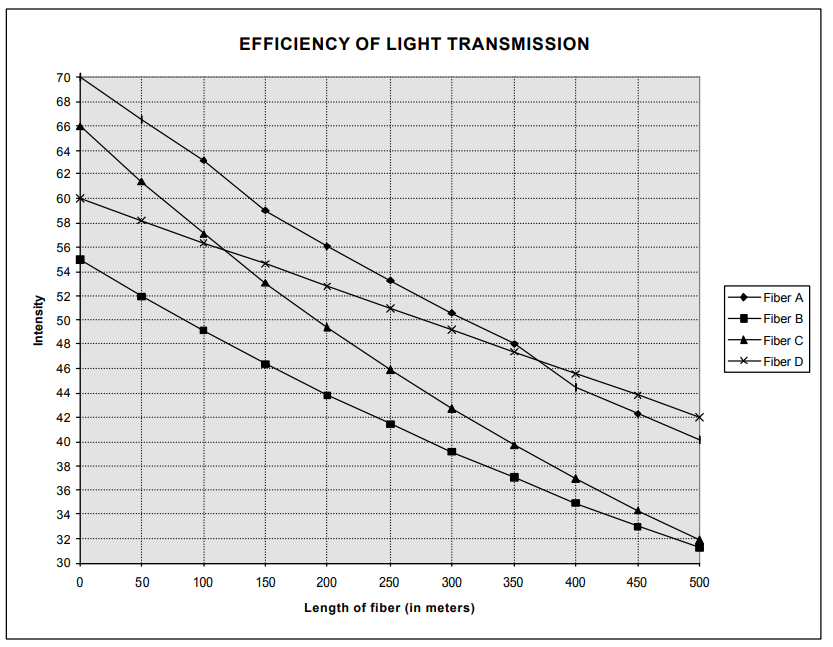Subject:
Mathematics

Topics: AlgebraExponential Equations and Functions
Common Core State Standard: A-SSE.3c,
Knowledge and Skills:

· Can determine the equation of an exponential function that closely matches a set of points

Lesson:

Procedure: This project should be done by students individually or in teams of two.

Distribute the handout and discuss it. Make sure that students understand what is represented by the four lines on the graph: how much light is transmitted through a fiber for varying distances.

Tell students you want them to try to determine, if possible, the equation for each of the graphs given, and make their recommendation as to the best fiber based on those equations.

Students should find that the equations for graphs B and C are both exponential. The equation for graph D is linear, and the equation for graph A will be very hard to determine.

Based on this it is likely that the data for fiber D is falsified. Of the remaining fibers, both A and C have the better transmission efficiencies (determined by taking the ratio of the output after 500 meters to the input light intensity). Fiber A, though, seems to have some inconsistencies as indicated by the non-smooth nature of the curve; therefore fiber C is the best choice.

To find the projected intensity at 1000 meters, students can solve the equation they generated. However, once they recognize that the curve is exponential they may realize that, by the nature of the exponential function, the intensity will drop by the same ratio over the second 500 meters as it did over the first. Thus the efficiency of transmission at 1000 meters is the square of the efficiency of transmission at 500 meters.

### Fiber

To: A.B.
From: T.W.

Alex,

I really appreciate your willingness to take some time out of your busy schedule to help me out on this fiber problem. Attached is the data that we’ve collected on efficiency of light transmission through four different brands of optical fibers.

As you can see in all cases the lab people measured the intensity of light that came through for various lengths of the fiber.

The trouble is that the data is coming from different sources so they didn’t all start with the same light intensity, which makes it harder to compare the fibers.

Also in at least one of these cases my researcher told me that he thought they just made up the data to make their fiber look good. But he didn’t say which one and he’s off on a three-week vacation in Samoa (without his cell phone!).

It will be a miracle if you can do anything with this, but I’m glad you will try.

The main thing I need to know is which brand of fiber is the best at transmitting light and seems to be the most reliable in its manufacturing process.

I’ll be happy to pay your outrageously high consultant fee if you can give me these answers.

Best,

Tom

P.S. For the fiber you recommend, I also need to know its efficiency at transmitting light over a 1000-meter distance. I’m sure that’s impossible but if you can somehow figure it out from this data I’ll double your fee.